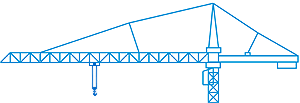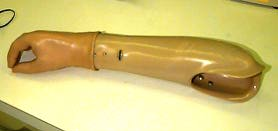# Master's and PhD Degrees  /  Courses  /  Introductory Soil MechanicsMECH_ENG 362: Stress Analysis

### Quarter Offered

Spring : MWF 10:00-10:50 ; Krishnaswamy

CIV ENG 216

### DescriptionTheory of elasticity: elastic stability, principal of minimum potential energy, Raylegh-Ritz methods. Introduction to finite element methods of stress analysis: computer implementation and use of commercial codes. Structural analysis of rods, beams, columns, and plates.

#### Who Takes It

Students interested in gaining a deeper appreciation of the mechanics of deformable solids than provided in the introductory Mechanics of Materials (Civ Eng 216) course are welcome to take this course. Typically students in mechanical engineering and biomedical engineering (biomechanics specialization) take this course in their junior or senior years.ME 362 provides a fundamental understanding of the mechanics of deformable solids. Starting with the basic concepts of stress and strain, the course walks you through several ways of analyzing structural systems: "exact" methods using the framework of the theory of elasticity; approximate methods using energy principles; and computational methods using finite element techniques.

#### Lectures

The course meets three days a week for 50-minute lectures. There is also a computer lab (times and dates announced during the first week of class).

#### Minisyllabus

• Characterization of Internal Forcesstress tensor; equations of equilibrium
• Characterization of the Geometry of Deformation
• displacements; strain tensor; compatibility
• Material Response:
• isotropic linear elastic behavior
• Theory of Elasticity• field equations of elasticity in three dimensions
• plane stress and plane strain
• Bernoulli-Euler Beam Bending Theory
• Principle of Minimum Potential Energy
• Rayleigh-Ritz Techniques• approximate methods of structural analysis
• The Finite Element Method
• principles
• ANSYS finite element software package
• Analysis of Structures:
• analysis of complex structures made of rods, beams, plates
• Elastic stability:buckling of columns

#### Labs

A computer lab where you learn to use the finite element package ANSYS